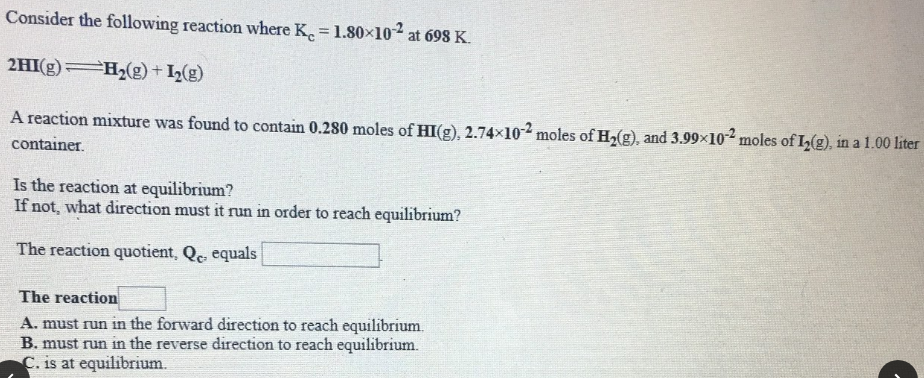# Consider the following reaction where Kc = 1.80x10^-2 at 698 K. 2HI(g) ⇌ H2(g) + I2(g) A reaction mixture was found to contain 0.280 moles of HI(g), 2.74x10^-2 moles of H2(g), and 3.99x10^-2 moles of I2(g), in a 1.00 liter container. Is the reaction at equilibrium? If not, what direction must it run in order to reach equilibrium? The reaction quotient, Qc, equals ____. The reaction ____.